Temperature characteristics of semiconductor diode | Статья в журнале «Молодой ученый»

Отправьте статью сегодня! Журнал выйдет 29 мая, печатный экземпляр отправим 2 июня.

Опубликовать статью в журнале
Проекты
Меню

Рубрика: Физика

Дата публикации: 12.05.2020

Статья просмотрена: 7 раз

### Библиографическое описание:

Temperature characteristics of semiconductor diode / Р. Р. Абдурахимов, Сарвар Азимов, А. А. Мирзаалимов [и др.]. — Текст : непосредственный // Молодой ученый. — 2020. — № 19 (309). — С. 85-89. — URL: https://moluch.ru/archive/309/69855/ (дата обращения: 15.05.2021).

﻿

In this article, describe temperature influence on silicon bases diode. Besides, give results that is taken by simulation.

Key words: semiconductor diode, model, p-n junction, temperature

In the context of global development, no device can be imagined without a semiconductor diode. That is, semiconductor diodes are widely used in various fields for various purposes. Semiconductor diodes are very sensitive to temperature, and their internal properties change very rapidly with changes in ambient temperature. This is due to the fact that the concentration of specific charge carriers in semiconductors increases sharply with increasing temperature. Suppose that the concentration of specific charge carriers increases, so that the recombination rate, the volt-ampere characteristic, the reverse current value, and a number of similar parameters change like a chain. This begs the question: is the effect of temperature beneficial? Of course not, because each device is designed for a nominal operating mode at the time of manufacture. Usually 300K is chosen for this .

As mentioned above, the temperature of the diode increases not only due to external influences, but also due to internal processes. An example of this is the recombination process. That is, in the reunion of electrons and holes, we can determine whether a photon forms a photon or a phonon by looking at the energy zones of the semiconductor. If it produces a photon and it can separate from the photon, such a diode is called a light emitting diode. Basically, such diodes are made of GaAs . That is, its energy field is direct. Silicon is indirect. This means that due to recombination, a phonon, not a photon, is released. A phonon is a quantum that oscillates the atoms of a crystal lattice. The energy of vibration can be equivalent to temperature. If we look closely, we can see in Figure 1 that there is a lot of recombination heat around the p-n junction. Which means it's about to be the most delusional time of the year, as well.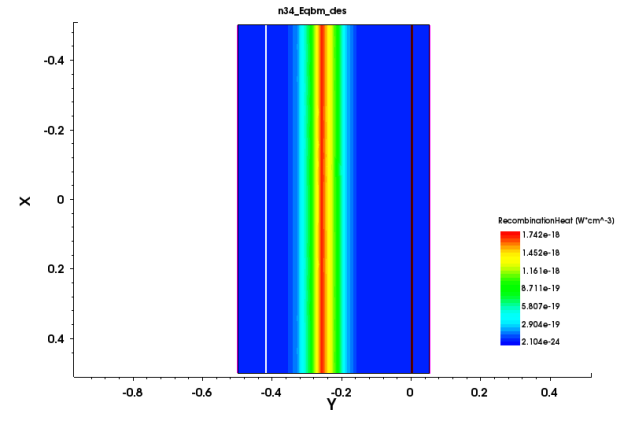Fig 1. Distribution of heat due to recombination in a p-n junction diode

Theoretically, the motion of electrons and holes should generate heat. This heat is called electron or hollow joule heat. I think every movement creates heat. Heat, on the other hand, makes any matter move. In the second figure above, the n-type electrons have a high amount of joule heat. Of course, we can explain this by the high concentration of electrons in the n-type. But that’s not the only reason. There are other reasons. That is, electrons in the n-type move faster than those in the p-type. The holes are similarly only inverted to electrons.

The model parameters of the p-n junction diode generated above are as follows:

Acceptor concentration: p = 1e16

Donor concentration: n = 1e19

T = 300 k

All the results obtained belong to this model.

Now that we understand the heat generated by a diode's internal processes, let's look at the direct effect of the external environment, that is, the effect of temperature on internal processes. Let’s check it in the simplest recombination.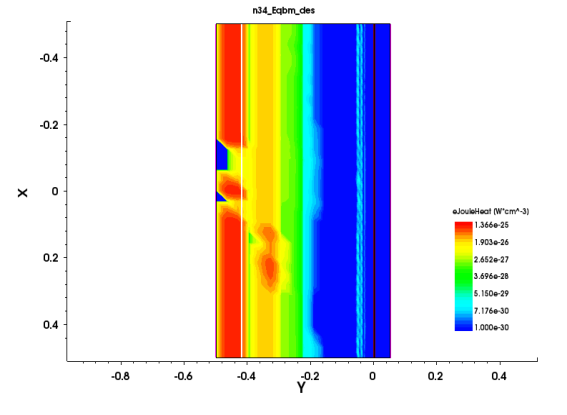Fig 2. The distribution of the amount of joule heat generated by the electrons in a p-n junction diode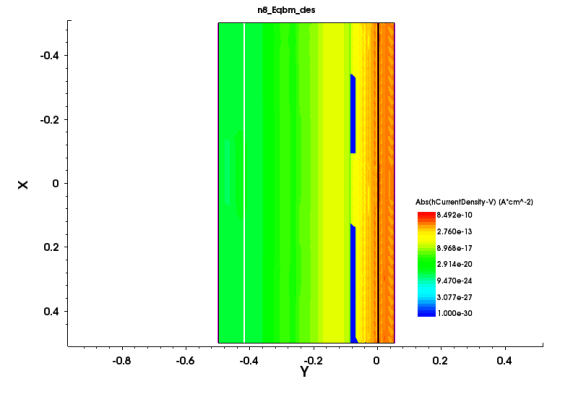Fig 3. The distribution of the amount of joule heat generated by the holes in a p-n junction diode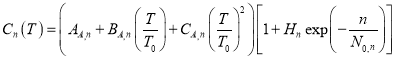(1)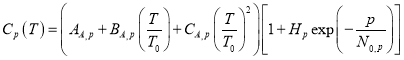(2)

Formulas auger recombination coefficients. As we can see, they are strongly related to temperature . The effect of temperature on the lifetime of electrons and holes in a diode can be calculated from the following expressions.(3)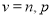(4)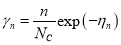(5)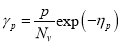(6)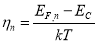(7)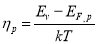(8)

The volt-ampere characteristic of a diode is also exponentially related to temperature .

In short, the effect of temperature on a semiconductor diode is very significant. As the temperature increases, the recombination rate also increases. This reduces the lifespan of electrons and choles. I can also conclude that through modeling we can increase the effectiveness of scientific research and understand events more fully.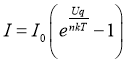(1)

Here:

I0 is the reverse current

n is the ideal coefficient, which is n = 2 in silicon-based diodes .

T is temperature.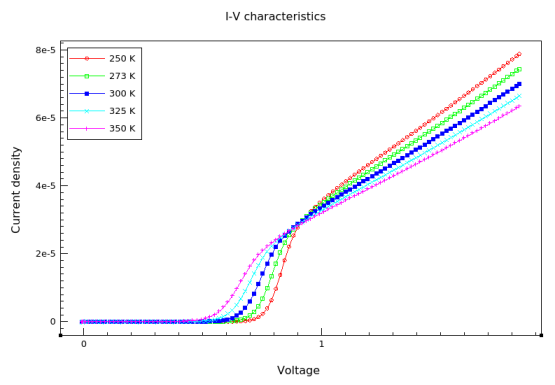Fig. 4. Volt-ampere characteristics of a p-n junction silicon diode at different temperatures

References:

1. Anderson and Anderson McGraw-Hill, “Fundametals of semiconductor devices”, 2016
2. M. Bashahu, P. Nkundabakura, «Review and tests of methods for the determination of the solar cell junction ideality factors», Solar Energy, vol. 81, pp. 856–863, July 2007.
3. J. Roulston, An Introduction to the Physics of Semiconductor
4. Devices, vol. 1, Oxford University Press, 1999

## Ключевые слова

model, temperature, semiconductor diode, p-n junction
Задать вопрос